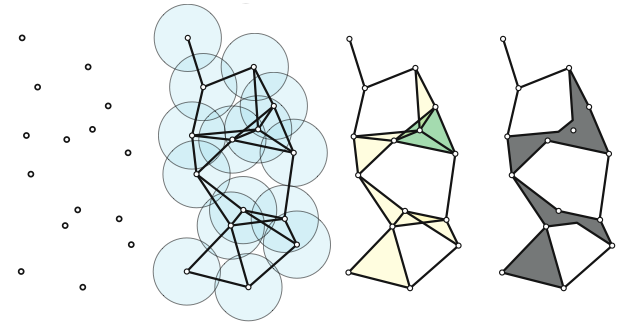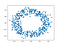# What is Topological Data Analysis?

## The Shape of DataPersistence Homology Pipeline: Data—→ ϵ balls —→ Vietoris Rips/Cech Complex — → Topological Features

# Introduction

Topological Data Science(TDA) has been bursting with new applications in machine learning and data science this year. The central dogma of TDA is that data (even complex, and high dimensional) has an underlying shape, and by understanding this shape we can reveal some kind of important information about the process which generate it. For a great survey on TDA for data scientists, check out . One of the ways which TDA helps understand this shape is through persistence homology, which will be briefly explained in this article. For actual computations, giotto-tda is a great toolkit for calculations and examples. The software is free, and instructions for downloading it are aviailable on the giotto-tda Github page along with several Jupyter notebooks highlighting some of the giotto-tda features.

# Persistent Homology

The goal of persistent homology is to determine the true topological descriptors of a dataset. As an intuitive motivation, suppose X a is a set of randomly generated points in two-dimensions, such as the one below.Point Cloud

Although real world data is much more complicated than this, it is helpful to understand how TDA applies to datasets which we already understand. We know that the data shown above, for example, is a single connected component with a single hole in the middle. Hence, we should be able to confirm this using persistent homology. But first, we need a few preliminary concepts from Algebraic Topology.

# Simplicial Complexes

Simplicial complexes are the main object of study in TDA. They are a topological space which can be thought of as higher dimensional graphs. They are composed by “ gluing together” multiple simplices.

More specifically, given a set X={x₁,…,xd} of affinely independent points in ℝᵈ, a k-dimensional simplex σ=[x₁,…,xₖ] is the convex hull of X.

(a) 0-simplex is a vertex, (b) 1-simplex is a line, (c )2-simplex is a triangle, (d) 3-simplex is a tetrahedron

Simplicies make up simplicial complexes, that is, in a very informal way, we can say that

I am avoiding mathematical rigor here by saying “glued together”, but I think it is more useful to understand what the general vibe is first. The picture below is an example of a simplicial complex with (18) 0-simplexes, (23) 1-simplexes, (8) 2-simplexes, and a single 3-simplex

Notice the way that simplices are glued together. They must be glued in a certain way. To show what I mean by this without getting too technical, consider the non-example shown below.

The motivation for defining simplicial complexes is that we can think of each one of our data points as a simplex, and thus by creating a simplicial complex from these simplices, we are able to extract meaningful topological descriptors which can reveal the shape of our dataset.
But how do know which points in our data represent which simplexes? And how do we actually obtain a simplicial complex?

It’s crucial to keep these questions in mind as we go along, so that we don’t get lost in the abstractions, and to make use of the definitons.

# The Čech Comple

Given a dataset X (a point cloud), we want to create a simplicial complex. To do this, the idea is to place a closed ball Bϵ(X)of radius ϵ around each point, and create an edge between any two points if their closed ϵ balls intersect.

Above we see the simplicial complex has 1 connected component, and 1 hole. But what if we change the value of ϵ? For example, if ϵis equal to the largest distance between any two points, then we obtain a single simplex.

The natural question to ask at this point, is what is the best choice for the value of ϵ? But we are not interested in answering this. Rather, we analyze the topological features which persist as we let ϵ vary (hence, the name persistence homology). The features which persist as ϵvaries greatly are considered to be the “true” features.

# Persistence Diagrams

The way that we record the topological features ( betti numbers) is by creating persistence diagrams. These diagrams record the birth time, and death time of a feature, with the birth time on the x axis, and the death time on the y axis. Traits which are closer to the diagonal usually represent noise (but this is not always the case, especially when applied to biological data), and traits further from the diagonal represent features that persist.

In the next article, we’ll take a look at some concrete examples and applications of Persistence. But that’s all for this one folks. Stay vibing, friends.

# References

1. Chazal, Frédéric, and Bertrand Michel. “An Introduction to Topological Data Analysis: Fundamental and Practical Aspects for Data Scientists.” ArXiv.org, 11 Oct. 2017, arxiv.org/abs/1710.04019.
2. https://en.wikipedia.org/wiki/Simplicial_complex
3. https://github.com/giotto-ai/giotto-tda

Originally published at https://jacobbriones1.github.io on November 8, 2020.

Specializing in explainable AI, mathematics, and physical sciences through the use of visualization, computer science, and creative writing.

## More from Jacob Briones

Specializing in explainable AI, mathematics, and physical sciences through the use of visualization, computer science, and creative writing.